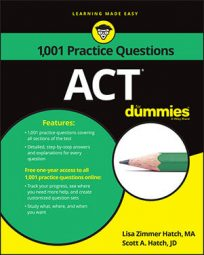##### ACTOkay, so "combinations and permutations" sounds like the name of a class you would take at wizards' college, but these are actually topics that you would cover in a statistics class. They're also something you'll probably need to know for the ACT Math exam.

Fortunately, the following practice questions will help you brush up on your skills: first, you'll need to calculate the total number of possible license plate designations for a community, and then you'll be asked to find the total possible number of combinations for a secret code.

## Practice questions

1. License plate designations in Tinytown consist of three characters. The first is either the letter M or F depending on the gender of the car's owner, the second is a single digit between 0 and 9, and the last is a single letter of the entire alphabet from A to Z. How many license plate designations are possible?

A. 38 B. 468 C. 520 D. 780 E. 6,760

2. A secret code is created by combining any 2 letters from the English alphabet and any 2 one-digit numbers between and including 0 and 9. How many different code combinations are possible if numeric digits can be repeated but letters cannot?

A. 71 B. 72 C. 60,840 D. 65,000 E. 67,600

1. The correct answer is Choice (C).

There are 2 choices for the first character (M or F), 10 choices for the second character (0, 1, 2, 3, 4, 5, 6, 7, 8, and 9), and the 26 letters of the alphabet for the third character. All you do is multiply the possibilities:

If you picked Choice (B), you thought there were 9 numbers from 0 to 9 instead of 10. Choice (A) results from incorrectly adding the numbers instead of multiplying them.

2. The correct answer is Choice (D).

Determine the total pool of elements you have to create code combinations. You can repeat numbers, and there are 10 separate digits from 0 to 9. Letters cannot be repeated, and there are 26 possibilities in the English alphabet. Apply the multiplication principle by multiplying the total possibilities for each element of the code. There are 10 for the first position, 10 for the second, 26 for the third, and 25 for the fourth (because you can't repeat the letter in the third position). The product of 10, 10, 26, and 25 is 65,000.